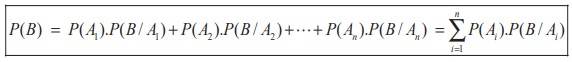Home | | Maths 11th std | Total Probability of an event

# Total Probability of an event

If A1 , A2 , A3 , ..., An are mutually exclusive and exhaustive events and B is any event in S then P(B) is called the total probability of event B.

Total Probability of an event

## Theorem 12.10 (Total Probability of an event)

If A1 , A2 , A3 , ..., An are mutually exclusive and exhaustive events and B is any event in S then P(B) is called the total probability of event B and## Proof

Since B is any event in S, from the figure shown here

B= ( A1 Ōł® B) Ōł¬ ( A2 Ōł® B) Ōł¬ ( A3 Ōł® B) Ōł¬ ...( An Ōł® B).

Since A1 , A2 , A3 ... An  are mutually exclusive,

A1 Ōł® B ) , ( A2 Ōł® B ) , ( A3 Ōł® B ) ,...,( An Ōł® B) are also mutually exclusive.

ThereforeThe following problems are solved using the law of total probability of an event.

## Example 12.24

Urn-I contains 8 red and 4 blue balls and urn-II contains 5 red and 10 blue balls. One urn is chosen at random and two balls are drawn from it. Find the probability that both balls are red.

## Solution

Let A1 be the event of selecting urn-I and A2 be the event of selecting urn-II.

Let B be the event of selecting 2 red balls.

We have to find the total probability of event B . That is, P(B).

Clearly A1 and A2 A1 are mutually exclusive and exhaustive events.## Example 12.25

A factory has two machines I and II. Machine-I produces 40% of items of the output and Machine-II produces 60% of the items. Further 4% of items produced by Machine-I are defective and 5% produced by Machine-II are defective. If an item is drawn at random, find the probability that it is a defective item.

## Solution

Let A1 be the event that the items are produced by Machine-I,   A2 be the event that items are produced by Machine-II. Let B be  the event of drawing a defective item.We have to find the total probability of event B . That is, P(B).

Clearly A1 and A2 are mutually exclusive and exhaustive events.

Therefore, P(B) = P (A1 ) . P (B / A1 ) + P (A2 ) . P (B / A2 )

We have P (A1 ) = 0.40, P (B / A1 ) = 0.04

P (A2 ) = 0.60, P (B / A2 ) =0.05

P(B) = P (A1 ) . P (B / A1 ) + P (A2 ) . P (B / A2 )

=( 0.40) ( 0.04) + ( 0.60 )( 0.05)

=0.046.

Tags : Theorem, Solved Example Problems | Probability Theory , 11th Mathematics : UNIT 12 : Introduction to Probability Theory
Study Material, Lecturing Notes, Assignment, Reference, Wiki description explanation, brief detail
11th Mathematics : UNIT 12 : Introduction to Probability Theory : Total Probability of an event | Theorem, Solved Example Problems | Probability Theory